Courses

# Introduction to Compressible Flow (Part - 2) Civil Engineering (CE) Notes | EduRev

## Civil Engineering (CE) : Introduction to Compressible Flow (Part - 2) Civil Engineering (CE) Notes | EduRev

The document Introduction to Compressible Flow (Part - 2) Civil Engineering (CE) Notes | EduRev is a part of the Civil Engineering (CE) Course Fluid Mechanics - Notes, Videos, MCQs & PPTs.
All you need of Civil Engineering (CE) at this link: Civil Engineering (CE)

Entropy and Second Law of Thermodynamics

• Equation (38.24) does not tell us about the direction (i.e., a hot body with respect to its surrounding will gain temperature or cool down) of the process. To determine the proper direction of a process, we define a new state variable, entropy, which is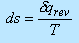(38.25)

where s is the entropy of the system,  δqrev is the heat added reversibly to the system and T is the temperature of the system. It may be mentioned that Eqn. (38.25) is valid if both external and internal irreversibilities are maintained during the process of heat addition

• Entropy is a state variable and it can be associated with any type of process, reversible or irreversible. An effective value of δqrev can always be assigned to relate initial and end points of an irreversible process, where the actual amount of heat added is δq . One can write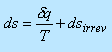(38.26)

It states that the change in entropy during a process is equal to actual heat added divided by the temperature plus a contribution from the irreversible dissipative phenomena. It may be mentioned that dsirrev implies internal irreversibilities if T is the temperature at the system boundary. If T is the temperature of the surrounding dsirrev implies both external and internal irreversibilities. The irreversible phenomena always increases the entropy, hence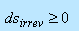(38.27)

• Significance of greater than sign is understandable. The equal sign represents a reversible process. On combining Eqs (38.26) and (38.27) we get(38.28)

If the process is adiabatic,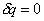, Eq. (38.28) yields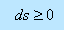(38.29)

• Equations (38.28) and (38.29) are the expressions for the second law of thermodynamics. The second law tells us in what direction the process will take place. The direction of a process is such that the change in entropy of the system plus surrounding is always positive or zero (for a reversible adiabatic process). In conclusion, it can be said that the second law governs the direction of a natural process.

• For a reversible process, it can be said that  δW = - pdv where dv is change in volume and from the first law of thermodynamics it can be written as

δq - pdv = de                                                 (38.30)

• If the process is reversible, we use the definition of entropy in the form  δqrev = Tds the Eq. (38.30) then becomes,
δq - pdv = de
Tds = de + pdv                                         (38.31)

• Another form can be obtained in terms of enthalpy. For example, by definition

h = e +pv

Differentiating, we obtain

dh = de + pdv + vdp                                  ( 38.32)

Combining Eqs (38.31) and (38.32), we have

Tds = dh + vdp                                  ( 38.33)

• Equations (38.31) and (38.33) are termed as first Tds equation and second Tds equation, respectively.

• For a thermally perfect gas, we have dh= cpdt  (from Eq. 38.20) , substitute this in Eq. (38.33) to obtain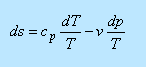( 38.34)

Further substitution of pv = RT into Eq. (38.34) yields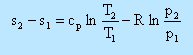( 38.35)

Integrating Eq. (38.35) between states 1 and 2,( 38.36)

If   cp  is a variable, we shall require gas tables; but for constant  cp  we obtain the analytic expression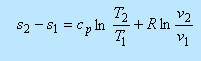( 38.37)

In a similar way, starting with Eq. (38.31) and making use of the relation  the change in entropy can also be written as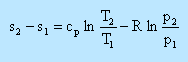( 38.38)

Isentropic Relation

An isentropic process is a reversible-adiabatic process. For an adiabatic process  δq = 0 and for a reversible process, dsirrev = 0 From Eq. (38.26), for an isentropic process, ds = 0 However, in Eq. (38.37), substitution of isentropic condition yields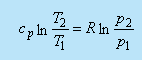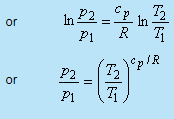(38.39)

Using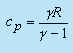, we have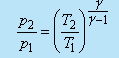(38.40)

Considering Eq. (38.38), in a similar way, yields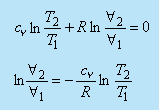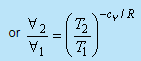(38.41)

Using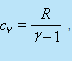we get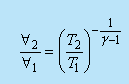(38.42)

• Using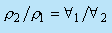we can write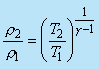(38.43)

• Combining Eq. (38.40) with Eq. (38.43), we find,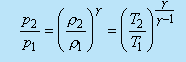(38.44)

This is a key relation to be remembered throughout the chapter.

Offer running on EduRev: Apply code STAYHOME200 to get INR 200 off on our premium plan EduRev Infinity!

,

,

,

,

,

,

,

,

,

,

,

,

,

,

,

,

,

,

,

,

,

;